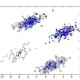# K-means clustering and comparison of K-means clustering algorithms in Python, Java and R

What is K-means Clustering:

The k-means algorithm is one of the simplest clustering techniques commonly used in data analytics.

Original Definition :

The k-means algorithm is an evolutionary algorithm that gains its name from its method of operation. The algorithm clusters observations into k groups, where k is provided as an input parameter. It then assigns each observation to clusters based upon the observation’s proximity to the mean of the cluster. The cluster’s mean is then recomputed and the process begins again.

A very Simple Example:

If you have a collection of dog and you would want to cluster them based on their gender then K =2 would be perfect.

A very complex Example:

If you have the same collection of dog and you would want to cluster them based on their size you could put K=5 (Extra Large, large, medium, small, extra small) however if you would use their family, you may be using K=50, may be cause you just only know 50 types of dog families and this may not be suitable scenario.

K-means clustering algorithms in Python:

#!/usr/bin/python
import sys
from math import fabs
from org.apache.pig.scripting import Pig

filename = “student.txt”
k = 4
tolerance = 0.01

MAX_SCORE = 4
MIN_SCORE = 0
MAX_ITERATION = 100

# initial centroid, equally divide the space
initial_centroids = “”
last_centroids = [None] * k
for i in range(k):
last_centroids[i] = MIN_SCORE + float(i)/k*(MAX_SCORE-MIN_SCORE)
initial_centroids = initial_centroids + str(last_centroids[i])
if i!=k-1:
initial_centroids = initial_centroids + “:”

P = Pig.compile(“””register udf.jar
DEFINE find_centroid FindCentroid(‘\$centroids’);
raw = load ‘student.txt’ as (name:chararray, age:int, gpa:double);
centroided = foreach raw generate gpa, find_centroid(gpa) as centroid;
grouped = group centroided by centroid;
result = foreach grouped generate group, AVG(centroided.gpa);
store result into ‘output’;
“””)

converged = False
iter_num = 0
while iter_num<MAX_ITERATION:
Q = P.bind({‘centroids’:initial_centroids})
results = Q.runSingle()
if results.isSuccessful() == “FAILED”:
raise “Pig job failed”
iter = results.result(“result”).iterator()
centroids = [None] * k
distance_move = 0
# get new centroid of this iteration, caculate the moving distance with last iteration
for i in range(k):
tuple = iter.next()
centroids[i] = float(str(tuple.get(1)))
distance_move = distance_move + fabs(last_centroids[i]-centroids[i])
distance_move = distance_move / k;
Pig.fs(“rmr output”)
print(“iteration ” + str(iter_num))
print(“average distance moved: ” + str(distance_move))
if distance_move<tolerance:
sys.stdout.write(“k-means converged at centroids: [“)
sys.stdout.write(“,”.join(str(v) for v in centroids))
sys.stdout.write(“]n”)
converged = True
break
last_centroids = centroids[:]
initial_centroids = “”
for i in range(k):
initial_centroids = initial_centroids + str(last_centroids[i])
if i!=k-1:
initial_centroids = initial_centroids + “:”
iter_num += 1

if not converged:
print(“not converge after ” + str(iter_num) + ” iterations”)
sys.stdout.write(“last centroids: [“)
sys.stdout.write(“,”.join(str(v) for v in last_centroids))
sys.stdout.write(“]n”)

K-means clustering algorithms in Java:

import java.io.IOException;

import org.apache.pig.EvalFunc;
import org.apache.pig.data.Tuple;
public class FindCentroid extends EvalFunc<Double> {
double[] centroids;
public FindCentroid(String initialCentroid) {
String[] centroidStrings = initialCentroid.split(“:”);
centroids = new double[centroidStrings.length];
for (int i=0;i<centroidStrings.length;i++)
centroids[i] = Double.parseDouble(centroidStrings[i]);
}
@Override
public Double exec(Tuple input) throws IOException {
double min_distance = Double.MAX_VALUE;
double closest_centroid = 0;
for (double centroid : centroids) {
double distance = Math.abs(centroid – (Double)input.get(0));
if (distance < min_distance) {
min_distance = distance;
closest_centroid = centroid;
}
}
return closest_centroid;
}

}

K-means clustering algorithms in R:

kmeans =
function(points, ncenters, iterations = 10, distfun = NULL) {
if(is.null(distfun))
distfun =
function(a,b) norm(as.matrix(a-b), type = ‘F’)
newCenters =
kmeans.iter(
points,
distfun,
ncenters = ncenters)
for(i in 1:iterations) {
newCenters = kmeans.iter(points, distfun, centers = newCenters)}
newCenters}

kmeans.iter =
function(points, distfun, ncenters = dim(centers), centers = NULL) {
from.dfs(mapreduce(input = points,
map =
if (is.null(centers)) {
function(k,v) keyval(sample(1:ncenters,1),v)}
else {
function(k,v) {
distances = apply(centers, 1, function(c) distfun(c,v))
keyval(centers[which.min(distances),], v)}},
reduce = function(k,vv) keyval(NULL, apply(do.call(rbind, vv), 2, mean))),
to.data.frame = T)}

1.